Bernoulli measure

A measure describing the independent repetition of an experiment with two possible outcomes, such as playing head and tail with a possibly biased coin. This is the simplest and most basic probabilistic scheme for which the fundamental theorems of probability theory, such as the weak and strong law of large numbers, the integral and local limit properties and the large deviation principle, were first and most easily proved.

The scheme can be generalized to experiments with possibly more than two outcomes and can be mathematically formalized in the following way. Given a countable setand a finite setwithelements, which can be identified with the set of the firstpositive integers if there are no special requirements, andnon-negative numbers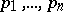with, one defines the corresponding Bernoulli measure on the spaceas a probability measure (finitely or countably additive, in accordance with the setting)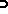such that the's are independent and identically distributed random variables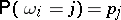for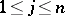. In the countable additive framework, a basic result is the Kolmogorov zero-one law [a9], stating that events in the algebra at infinity (i.e., events that are measurable with respect to the Borel fields generated by the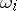's in the complement of every finite subset of) have probability either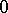or.

Percolation theory on Bernoulli measures is a theory that, although in a simpler setting, presents in many respects the same kinds of phenomena as statistical mechanics, with important difficult results and many open problems (see Statistical mechanics, mathematical problems in; [a8], [a3]). In the most common setting, one considers on each siteof a lattice a random variable with valuesand; such variables are assumed to be independent and identically distributed, so that one finds that the Bernoulli measures parametrized by the probabilityfor anare equal to. Many events considered in percolation theory are increasing (or positive) in the sense that their indicator functions are non-decreasing functions of the's. L. Russo [a7] has proved a finite version of Kolmogorov's zero-one law; it states that increasing events that in a suitable sense depend little on eachhave probability close toorfor all but a small interval of's. T.E. Harris [a4] has proved a basic inequality for increasing events on Bernoulli measures: ifandare increasing events, then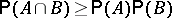. An inequality in the opposite sense was proved by J. van den Berg and H. Kesten [a1] for increasing events; it was generalized to arbitrary events by D. Reimer [a6]. It states that the probability that two events happen disjointly (in the sense that one can verify their occurrence by looking at disjoint subsets of the lattice) is less than or equal to the product of their probabilities.

The De Finetti theorem on infinite sequences of exchangeable random events [a2] shows that Bernoulli measures are relevant for statistical inference in a wide range of situations. It states that if the distribution of an infinite family of random events is invariant under finite permutations, then it can be expressed as a mixture of Bernoulli measures. The assumption of exchangeability is very natural in many concrete situations.

Let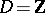be the set of integer numbers and letbe the left shift operator on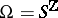: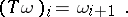The pair consisting of the Bernoulli measure and the shift operator is called the Bernoulli shift. One says that an invertible measurable mapping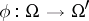between two Bernoulli shifts, with probability measuresandand shift operatorsand, is an isomorphism between Bernoulli shifts ifand. The famous Ornstein theorem [a5], which has been generalized in many ways, states that two Bernoulli shifts are isomorphic if and only if their Kolmogorov–Sinai entropies are equal. For a Bernoulli shift the Kolmogorov–Sinai entropy is given by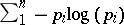, with the convention that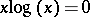for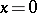.